#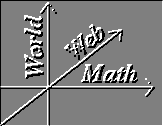Gradients

Prequisites: Partial Derivatives, Vectors

Let f(x,y,z) be a three-variable function defined throughout a region of three dimensional space, that is, a scalar field and let P be a point in this region. Say we move away from point P in a specified direction that is not necessarily along one of the three axes. How can we calculate the changes in f as we do this? What we want to calculate is the directional derivative

Well, let's start by letting R=x0i+y0j+z0k be the position vector for P. Let the specified direction that we want to move away from P be given by the unit vector u = u1i + u2j + u3k. Let Q=(x +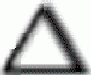x, y +y, z +z) be a point along the vector in the specified direction. Lets be the scalar value such that, that iss is the length of. Then.

##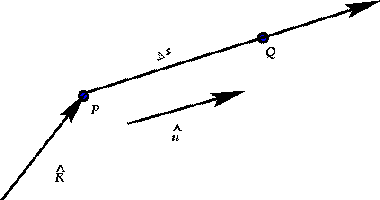Letf = f(Q) - f(P). By linear approximation,f = fx(P)x + fy(P)y + fz(P)z + erfx(Q)x + erfy(Q)y + erfz(Q)zf = fx(P)(s)u1 + fy(P)(s)u2 + fz(P)(s)u3 + erfx(Q)(s)u1 + erfy(Q)(s)u2 + erfz(Q)(s)u3 Dividing bys, we have,Now as Q approaches P along the line defined by the two points, the three error functions will go to zero. We get the directional derivative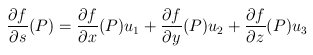Or, the dot product,where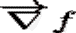is a special function defined as follows,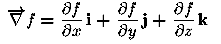The inverted Delta symbol and arrow ofis called the "Del Operator." Many texts will omit the vector arrow, which is also a faster way of writing the symbol. But the vector arrow is helpful to remind you that the gradient of a function produces a vector.

What we have just walked through is the explanation of the gradient theorem.

The Gradient Theorem: Let f(x,y,z), a scalar field, be defined on a domain D in R3. Assume that f(x,y,z) has linear approximations on D (i.e. is continuous on D)Then at each point P in D,

1. there exists a vector, such that for each direction u at P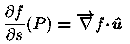2. the vectoris given by,This vector is called the gradient at P of the scalar field f. Another notational form ofis grad f. The directional derivative in any given direction is the scalar component of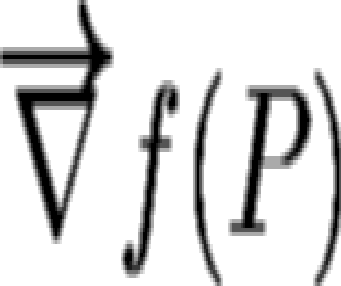in that direction.

So, for a point P of our function f, we have a vector defined. What is the geometrical meaning of this vector?

• The direction ofis the direction for whichhas the maximum value.
• The magnitude ofis that maximum value.
From the formula, we can see that the value ofvaries in a very smooth way as and that if u is orthogonal to, thenmust be 0.

Why is that? Lets try to get a visual conception. To do that lets think of a two-variable function instead of three-variable so that we can visualize a surface. We remember from calculus that when you look close enough at a curve (assuming it is continuous) that a small segment of it looks like straight line. Well, if we have a continuous surface, and if we look close enough at a little spot of it, it will look like a flat plane. We can think of a little spot of it as a tiny flat circle, (Note that in the past we have visualized each tiny section as a flat rectangle, but to understand gradients, a circle makes much more sense).The green circles are the area swept out by the vectors produced by the function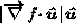, that is the vector from our point to the edge of the green circle has a length equal to the directional derivative in that direction. From the green circles you can see that as the direction approaches the vector perpendicular to the gradient, the directional derivative goes to zero.

Visualizing this concept in four-dimensional space is a bit more complex. But the gradient vector still points in the direction of greatest increase of the function and any vector perpendicular to the gradient will have a zero directional derivative. But in the past we have had a name for that surface intersecting a point on which f'=0. We called it the level surface. So we can make this statement.

The gradient of a function f(x,y,z) at a point P is normal to the level surface of f that passes through P

One thing we have not brought up yet but that ought to be tickling your curiousity is what the meaning is ofwhen a point has not been plugged into function. Clearly, a specific point does not need to be thought of to calculate. The first thing to note is thatis NOT a scalar function. Which meansis not like any function you've ever dealt with before most likely. Instead of producing a scalar value, it produces a vector. So what sort of space is created by this function that takes in three variables and spits out vectors? This space is called a vector field and will be the subject of the next section.

## Example

Now let's calculate a gradient. Lets let f(x,y,z)=x2y3 + zcosx at the point P=(pi/2,2,3). First we find the partial derivatives.

•••so,Now we plug in P.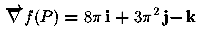Now what is the directional derivative along the vector v =i+2j-k from P? We need to find the dot product between the gradient of f at P and the unit vector in the direction of v. The unit vector along v is computed by scaling v by the reciprocal of its magnitude. |v|=(6)1/2, So the unit vector is.

Now we computeAnd this is the value of the direction derivative in the directio of vector v.

### Exercises:

1. Show that the gradient has the following properties, that demonstrate its similarity to the differentiation.
1.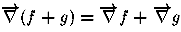2.3.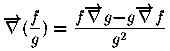4.Vector Calculus Index | World Web Math Main Page

thing@athena.mit.edu